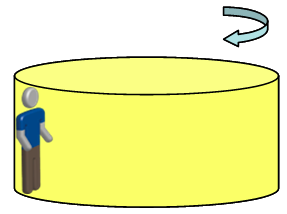# Problem: In a classic carnival ride, patrons stand against the wall in a cylindrical-shaped room. Once the room gets spinning fast enough, the floor drops from the bottom of the room! Friction between the walls of the room and the people on the ride me them the “stick” to the wall so they do not slide down. In one ride, the radius of the cylindrical room is R = 7.1 m and the room spins with a frequency of 21 revolutions per minute.1) What is the speed of a person “stuck” to the wall?2) What is the normal force of the wall on a rider of m = 51 kg?3) What is the minimum coefficient of friction needed between the wall and the person?4) If a new person with mass 102 kg rides the ride, what minimum coefficient of friction between the wall and the person would be needed?5) Which of the following changes would decrease the coefficient of friction needed for this ride?a) increasing the rider’s massb) increasing the radius of the ridec) increasing the rotation rate of rided) increasing the acceleration due to gravity (taking the ride to another planet)6) To be safe, the engineers making the ride want to be sure the normal force does not exceed 1.9 times each persons weight - and therefore adjust the frequency of revolution accordingly. What is the minimum coefficient of friction now needed?

###### FREE Expert Solution

In this problem, the normal force is:

$\overline{){\mathbf{N}}{\mathbf{=}}\frac{\mathbf{m}{\mathbf{v}}^{\mathbf{2}}}{\mathbf{R}}}$, where m is the mass of the rider, v is the speed of the rider, and R is the radius of the room.

Speed of the rider:

$\overline{){\mathbf{v}}{\mathbf{=}}{\mathbf{\omega }}{\mathbf{R}}}$

Angular frequency, ω = 21 revolutions per minute

Converting to rad/s:

ω = 2.199 rad/s

100% (130 ratings)###### Problem Details

In a classic carnival ride, patrons stand against the wall in a cylindrical-shaped room. Once the room gets spinning fast enough, the floor drops from the bottom of the room! Friction between the walls of the room and the people on the ride me them the “stick” to the wall so they do not slide down. In one ride, the radius of the cylindrical room is R = 7.1 m and the room spins with a frequency of 21 revolutions per minute.1) What is the speed of a person “stuck” to the wall?

2) What is the normal force of the wall on a rider of m = 51 kg?

3) What is the minimum coefficient of friction needed between the wall and the person?

4) If a new person with mass 102 kg rides the ride, what minimum coefficient of friction between the wall and the person would be needed?

5) Which of the following changes would decrease the coefficient of friction needed for this ride?
a) increasing the rider’s mass
b) increasing the radius of the ride
c) increasing the rotation rate of ride
d) increasing the acceleration due to gravity (taking the ride to another planet)
6) To be safe, the engineers making the ride want to be sure the normal force does not exceed 1.9 times each persons weight - and therefore adjust the frequency of revolution accordingly. What is the minimum coefficient of friction now needed?

Frequently Asked Questions

What scientific concept do you need to know in order to solve this problem?

Our tutors have indicated that to solve this problem you will need to apply the Conservation of Energy with Rotation concept. You can view video lessons to learn Conservation of Energy with Rotation. Or if you need more Conservation of Energy with Rotation practice, you can also practice Conservation of Energy with Rotation practice problems.